This video was brought to you by GeneralPAC.com, making power systems Intuitive, Open and Free for Everyone, Everywhere. Consider subscribing and supporting through patreon.com/GeneralPAC. This is a mechanism for you to support us financially so we can continue making high quality power system video tutorials. Our corporate sponsor for this topic is AllumiaX.com from Seattle, Washington. Contact them for industrial and commercial power system studies.

Introduction to the Delta Wye Transformer connection. Part 5b.

Okay so we're going to take these phasors and subtract phase A current with phase C current and then look at what our line current A looks like.

Alright. So here is phase A current and here is phase C current. Now if we want to perform phase A current plus phase C current, we would do this through what we call the head to tail method. And how that works is very simple. We would pick up phase C current and add it to the head of phase A current. And the resultant vector starts from the origin and go towards the head of phase C current. So this right here is our resultant vector. And this resultant vector equals phase A current plus phase C current.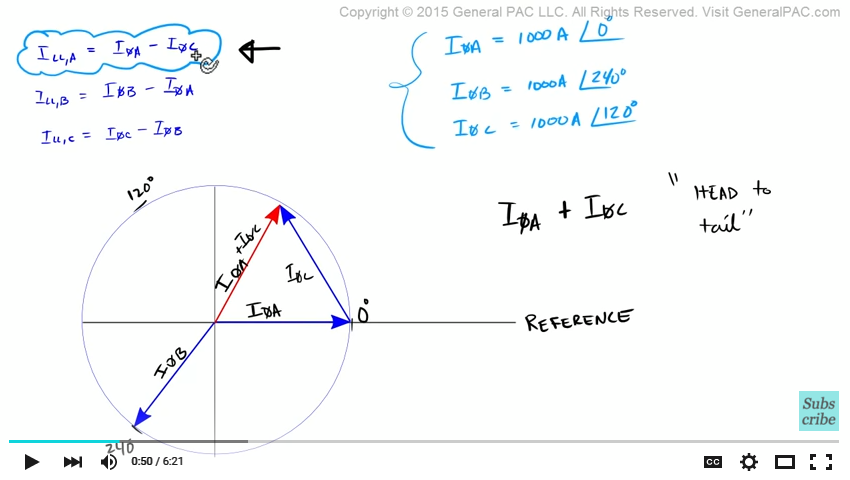However, this is not what we want. What we want to equate is this equation. Phase A current minus phase C current. So let's clean this up a little bit and put phase C current back to where it was.

Okay because there is a minus sign that's associated with the Phase C current, we have to pick up phase C current and rotate it 180 degrees. So phase C current becomes like this. So this phasor here is negative phase C current. So now, let's pick this up again and connect it to the head of phase A current so now we're going to connect it like that.

Okay. Now the resultant vector starts from the origin and ends at the head of negative phase C current. Now notice that the resultant vector longer now right? And the resultant vector represents phase A current minus phase C current.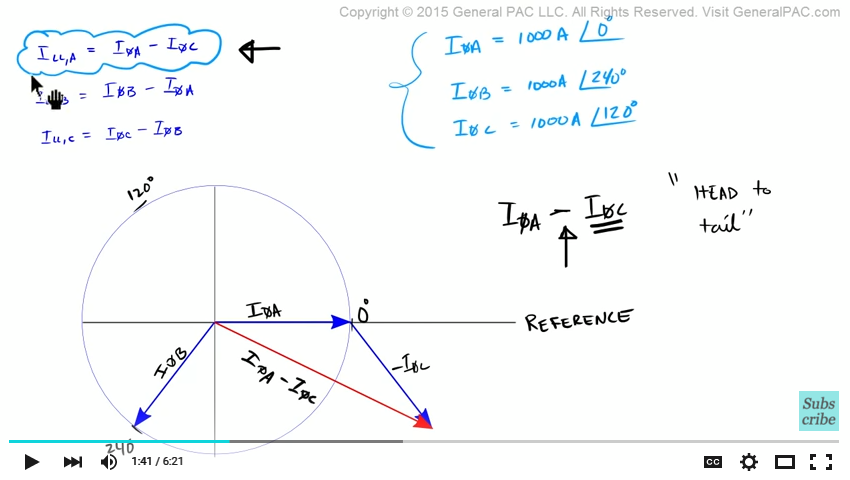Now believe it or not, this phasor diagram actually represents this particular equation alright. And this resultant vector is actually representing this line current here. So what we're saying when comparing line current with phase current A.

Now remember, this is phase current A here and this is line current here. So let's label that a little better. We see that the line current is a lot larger than our phase current right? This is our phase current here and this is our line current. So the magnitude of our line current which starts from the origin and ends at this tip here that is a lot larger than our phase current.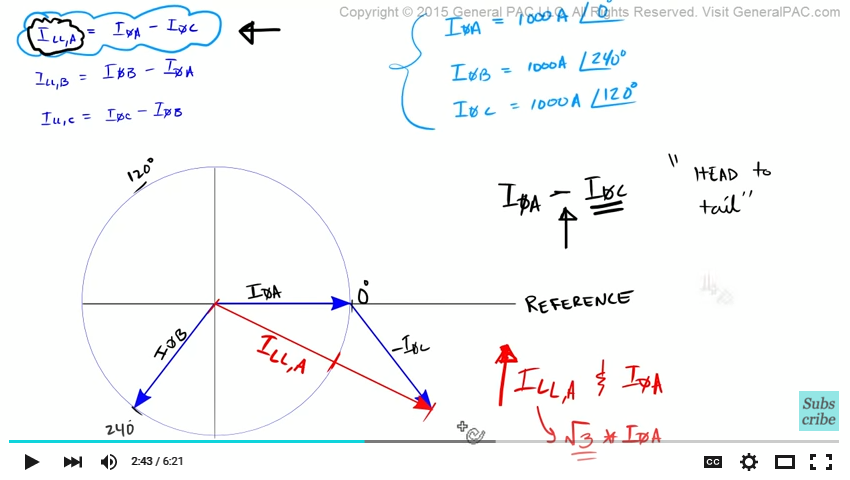In fact, if we compare the two, we will find that the line current is actually the square root of 3 times larger than our phase current.  So if we take our phase current here and line it up with our line current, we would be at this point here. But then we would have to stretch it or we would have to multiply it, by the square root of 3 or 1.73 to make it equal to our line current.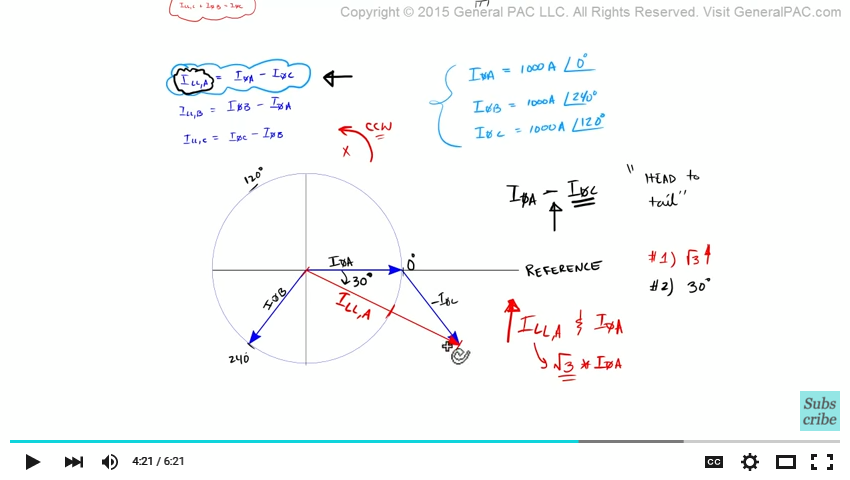So that's the first observation. That our line current is the square root of 3 times larger than our phase current. Okay. Now, also notice that our line current has a phase shift associated with it. Alright? So if all the phasors were rotating in the counter clockwise direction which they should according to international standards, they were all rotating in the counter clockwise direction, we will see that the line current has a 30 degrees phase shift okay.

So number 2, line current has a 30 degree phase shift with respect to phase current. Before we conclude here, when I was first learning this, this particular phasor analysis was very complicated. It didn't sit with me very well.

So let's take a step back and walk through this step by step. So what we're actually doing with this phasor analysis is we're trying to compute this particular equation. And what we're saying is if we subtract phase current A minus phase current C, we'll get line current A. And this is exactly what we did.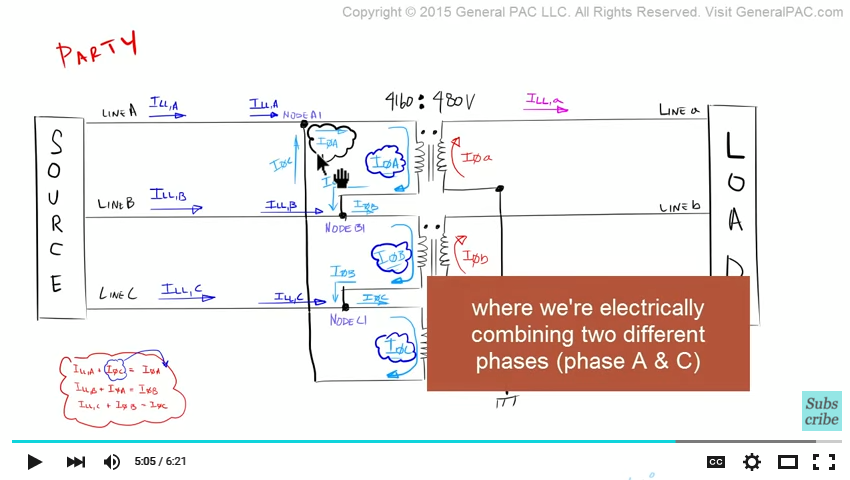We took phase current A and we subtracted phase current C and what we got right here is line current A. Okay. And all we're saying is that if we compare phase current A, which is this phasor here… with line current A which is this phasor here, we'll see that the line current is a lot larger than our phase current. Not only is it larger, but it also has a 30 degrees phase shift. This line current has a 30 degree phase shift.

So going back to our phasor diagram, what we're saying is that this line current here, if we compare this line current with this phase current. Which is the phase current that's flowing through the primary phase A winding.

This line current is going to be square root of 3 times larger than this phase current. And this line current is also going to have a 30 degree phase shift with this phase A current. Okay?

So let's take another step back and ask ourselves, why is our line current different from our phase current? Besides the fact that we have a delta connection. And we're electrically combining two different phase (phase A and Phase C) currents. Is because, when we set our phase A, B, and C current, we said that we would have a balanced system. And in this balanced system, we have 120 degree phase shift between our three phasors.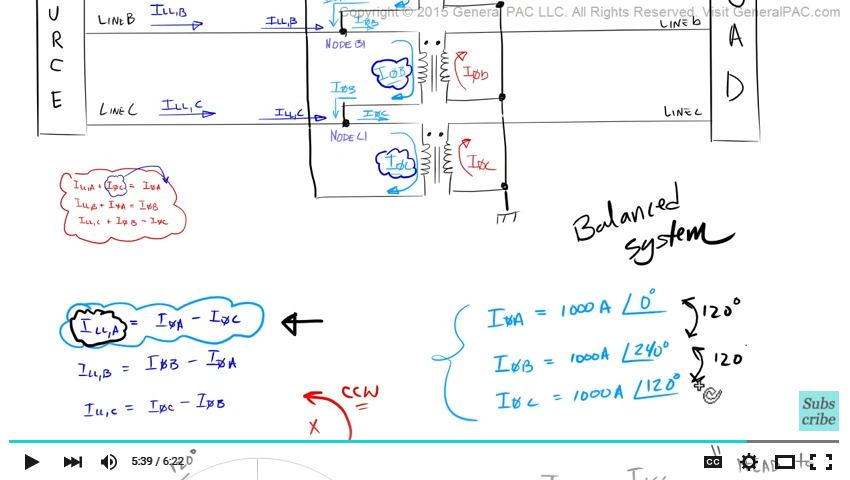So phase A is 120 degree apart from phase B. And Phase B is 120 degrees apart from phase C. Now because, we have a balanced system, and because the phase currents are a 120 degrees apart, when we're subtracting one phase current with another phase current, the angle is the magic that get's you the square root of 3 times larger and the 30 degree phase shift.

So it's the angle of phase A current which is at 0 degrees right? Which we defined here. And the angle of phase C current, which is at 120 degrees which we defined here. That's what's causing the square root of 3 times different and the 30 degrees phase shift between the phase current and the line current.

This video was brought to you by GeneralPAC.com. Making power system protection, automation, and controls Intuitive.

#### Greetings from the GeneralPAC Team!

We make high-quality Power Systems Video Tutorials on complex topics that are free and open to everyone!  Thank you so much for supporting us through Patreon so we can continue doing good and valuable work.

What is Patreon and why do we use it?

Patreon is a fantastic portal that allows our fans and community to make monthly contribution (like Netflix subscription) so we can continue creating high-quality power systems video tutorials. In return, you get access to incredible perks like voting on future topics, getting your questions answered, access to VIP Q/A webinars with the creators of GeneralPAC, and much more! We THANK YOU for supporting us

Why do we need your support?

An incredible amount of time and effort is needed to develop high-quality video tutorials. Each video (Part 1 for example) takes approximately 10 hours to complete which includes learning the concept ourselves, brainstorming creative ways to teach and explain the concepts, writing the script, audio recording, video recording, and editing. It's no wonder why Hundreds-of-Thousands of people have watched, liked, subscribed, and left positive comments on Youtube channel. Your support truly makes all the difference.